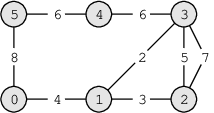Your browser (Unknown 0) is no longer supported. Some parts of the website may not work correctly. Please update your browser.

#### AscendingPaths

Find the longest path in a weighted graph in which the weights are ascending.

You are visiting the Royal Botanical Gardens. In the gardens there are N intersections connected by M roads. The intersections are numbered from 0 to N − 1. Each road connects two intersections and has an attractiveness level, which denotes how beautiful the plants along it are considered to be. The attractiveness level is a positive integer; the greater the better.

You are given a map of the gardens in the form of integer N and three arrays A, B and C. Each of the arrays contains M integers.

• For each I (0 ≤ I < M) there is a road between intersections A[I] and B[I] with an attractiveness level of C[I].
• There can be multiple roads connecting the same pair of intersections, or a road with both ends entering the same intersection.
• It is possible for roads to go through tunnels or over bridges (that is, the graph of intersections and roads doesn't have to be planar).

You want to find a walk in the gardens which will be as long as possible. You may start and finish at any intersections, and you may visit an intersection more than once during the walk. However, the walk must be ascending: that is, the attractiveness level of each visited road must be greater than that of the previously visited road.

Write a function:

class Solution { public int solution(int N, int[] A, int[] B, int[] C); }

that, given an integer N and arrays A, B and C of M integers, returns the maximal number of roads that can be visited during an ascending walk in the gardens.

For example, given N = 6 and the following arrays:

A = 0 B = 1 C = 4 A = 1 B = 2 C = 3 A = 1 B = 3 C = 2 A = 2 B = 3 C = 5 A = 3 B = 4 C = 6 A = 4 B = 5 C = 6 A = 5 B = 0 C = 8 A = 3 B = 2 C = 7the function should return 4. The longest ascending walk visits intersections 3, 1, 2, 3 and 4 (in that order). The attractiveness levels of the visited roads are 2, 3, 5 and 6, respectively.

Write an efficient algorithm for the following assumptions:

• N is an integer within the range [1..200,000];
• M is an integer within the range [0..200,000];
• each element of arrays A and B is an integer within the range [0..N-1];
• each element of array C is an integer within the range [1..1,000,000,000].